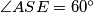### MEMO 2011 ekipno problem 5

Kvaliteta:
Avg: 3.5
Težina:
Avg: 6.0
Let$ABCDE$ be a convex pentagon with all five sides equal in length. The diagonals$AD$ and$EC$ meet in$S$ with$\angle ASE = 60^\circ$. Prove that$ABCDE$ has a pair of parallel sides.
Source: Srednjoeuropska matematička olimpijada 2011, ekipno natjecanje, problem 5